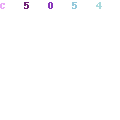# Yapımlar

######jQuery(document).ready(function() { jQuery('#sample_slider').owlCarousel({ items : 1, animateOut : 'fadeOut', smartSpeed : 300, autoplay : true, autoplayTimeout : 3500, smartSpeed : 300, fluidSpeed : 300, autoplaySpeed : 300, navSpeed : 300, dotsSpeed : 300, loop : true, autoplayHoverPause : true, nav : true, navText : ['',''], dots : true, responsiveRefreshRate : 200, slideBy : 2, mergeFit : true, mouseDrag : true, touchDrag : true }); sa_resize_sample_slider(); window.addEventListener('resize', sa_resize_sample_slider); function sa_resize_sample_slider() { var min_height = 'aspect169'; var win_width = jQuery(window).width(); var slider_width = jQuery('#sample_slider').width(); if (win_width < 480) { var slide_width = slider_width / 1; } else if (win_width < 768) { var slide_width = slider_width / 1; } else if (win_width < 980) { var slide_width = slider_width / 1; } else if (win_width < 1200) { var slide_width = slider_width / 1; } else if (win_width < 1500) { var slide_width = slider_width / 1; } else { var slide_width = slider_width / 1; } slide_width = Math.round(slide_width); var slide_height = '0'; if (min_height == 'aspect43') { slide_height = (slide_width / 4) * 3; slide_height = Math.round(slide_height); } else if (min_height == 'aspect169') { slide_height = (slide_width / 16) * 9; slide_height = Math.round(slide_height); } else { slide_height = (slide_width / 100) * min_height; slide_height = Math.round(slide_height); } jQuery('#sample_slider .owl-item .sa_hover_container').css('min-height', slide_height+'px'); } });